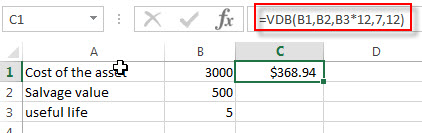# Excel VDB Function

This post will guide you how to use Excel VDB function with syntax and examples in Microsoft excel.

### Description

The Excel VDB function calculates the depreciation of an asset for a specified period based on the double declining balance method. And it will return a numeric value.

The VDB function is a build-in function in Microsoft Excel and it is categorized as a Financial Function.

The VDB function is available in Excel 2016, Excel 2013, Excel 2010, Excel 2007, Excel 2011 for Mac.

### Syntax

The syntax of the VDB function is as below:

`= VDB (cost, salvage, life, start_period, end_period, [factor], [no_switch])`

Where the VDB function arguments are:

• Cost -This is a required argument. The initial cost of the asset.
• Salvage -This is a required argument. The value of the asset at the end of the depreciation.
• Life -This is a required argument. The number of periods over which the asset is to be depreciated.
• Start_period -This is a required argument. The starting period for which you want to calculate the depreciation.
• End_period -This is a required argument. The ending period for which you want to calculate the depreication.
• Factor -This is an optional argument. It is specified the rate of depreciation.
• No_switch -This is a required argument. A logical value specifying whether to switch to straight-line depreciation when depreciation is greater than the declining balance calculation. And it can be either a value of TRUE or FALSE.
 TRUE Using declining balance method of depreciation. FALSE Using the straight-line depreciation method when the straight-line depreciation is greater than the declining balance depreciation amount.

### Excel VDB Function Examples

The below examples will show you how to use Excel VDB Function to calculate the straight line depreciation of an asset for one period.

#1 to get the depreciation between the 7th and 12th month for an asset that cost 3000, with a salvage value of 500, and the useful life of the asset is 5, using the following formula:

`=VDB(B1,B2,B3*12,7,12)`### Related Functions

• Excel SLN Function
The Excel SLN function calculates the depreciation of an asset for one period based on the straight line depreciation. And it will return a numeric value.The syntax of the SLN function is as below:= SLN (cost, salvage, life)…
• Excel SYD Function
The Excel SYD function calculates the sum-of-years’digits depreciation of an asset for a specified period. And it will return a numeric value.The syntax of the SYD function is as below:=SYD(cost, salvage, life, per)…

Related Posts

Excel DVAR Function

This post will guide you how to use Excel DVAR function with syntax and examples in Microsoft excel. Description The Excel DVAR Function will get the variance of a population based on a sample of numbers in a column in ...

Excel DSUM Function

This post will guide you how to use Excel DSUM function with syntax and examples in Microsoft excel. Description The Excel DSUM Function will add the numbers in a column or database that meets a given criteria. And so you ...

Excel DSTDEVP Function

This post will guide you how to use Excel DSTDEVP function with syntax and examples in Microsoft excel. Description The Excel DSTDEVP returns the standard deviation of a population based on the entire population of numbers in a column or ...

Excel DSTDEV Function

This post will guide you how to use Excel DSTDEV function with syntax and examples in Microsoft excel. Description The Excel DSTDEV returns the standard deviation of a population based on a sample of numbers in a column or database ...

Excel DMIN Function

This post will guide you how to use Excel DMIN function with syntax and examples in Microsoft excel. Description The Excel DMIN returns the minimum value from a database or a column of a list that matches the specified conditions. ...

Excel DPRODUCT Function

This post will guide you how to use Excel DPRODUCT function with syntax and examples in Microsoft excel. Description The Excel DPRODUCT returns the product of values from a set of records that match criteria that you specify. The DPRODUCT ...

Excel XIRR Function

This post will guide you how to use Excel XIRR function with syntax and examples in Microsoft excel. Description The Excel XIRR function returns the internal rate of return for a series of cash flows that is not necessarily periodic. ...

Excel SYD Function

This post will guide you how to use Excel SYD function with syntax and examples in Microsoft excel. Description The Excel SYD function calculates the sum-of-years’digits depreciation of an asset for a specified period. And it will return a numeric ...

Excel SLN Function

This post will guide you how to use Excel SLN function with syntax and examples in Microsoft excel. Description The Excel SLN function calculates the depreciation of an asset for one period based on the straight line depreciation. And it ...

Excel PV Function

This post will guide you how to use Excel PV function with syntax and examples in Microsoft excel. Description The Excel PV function returns the present value of a loan or investment based on constant payments and a constant interest ...

Sidebar